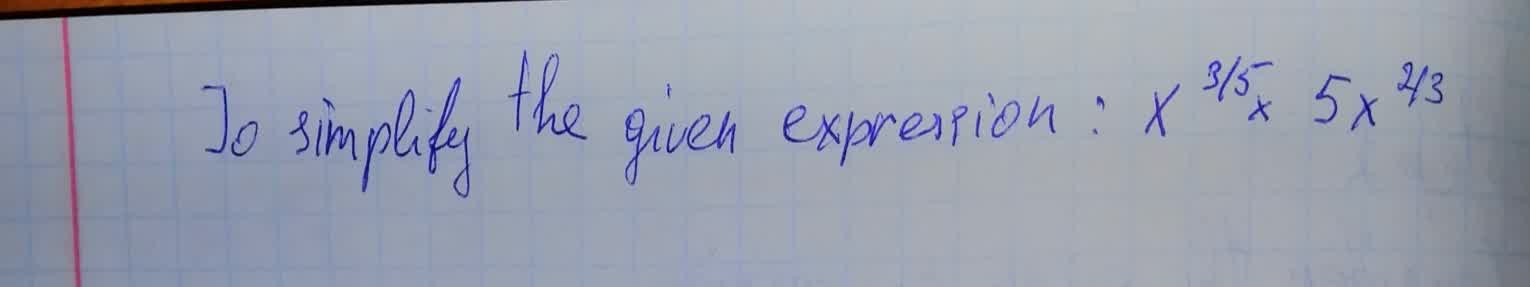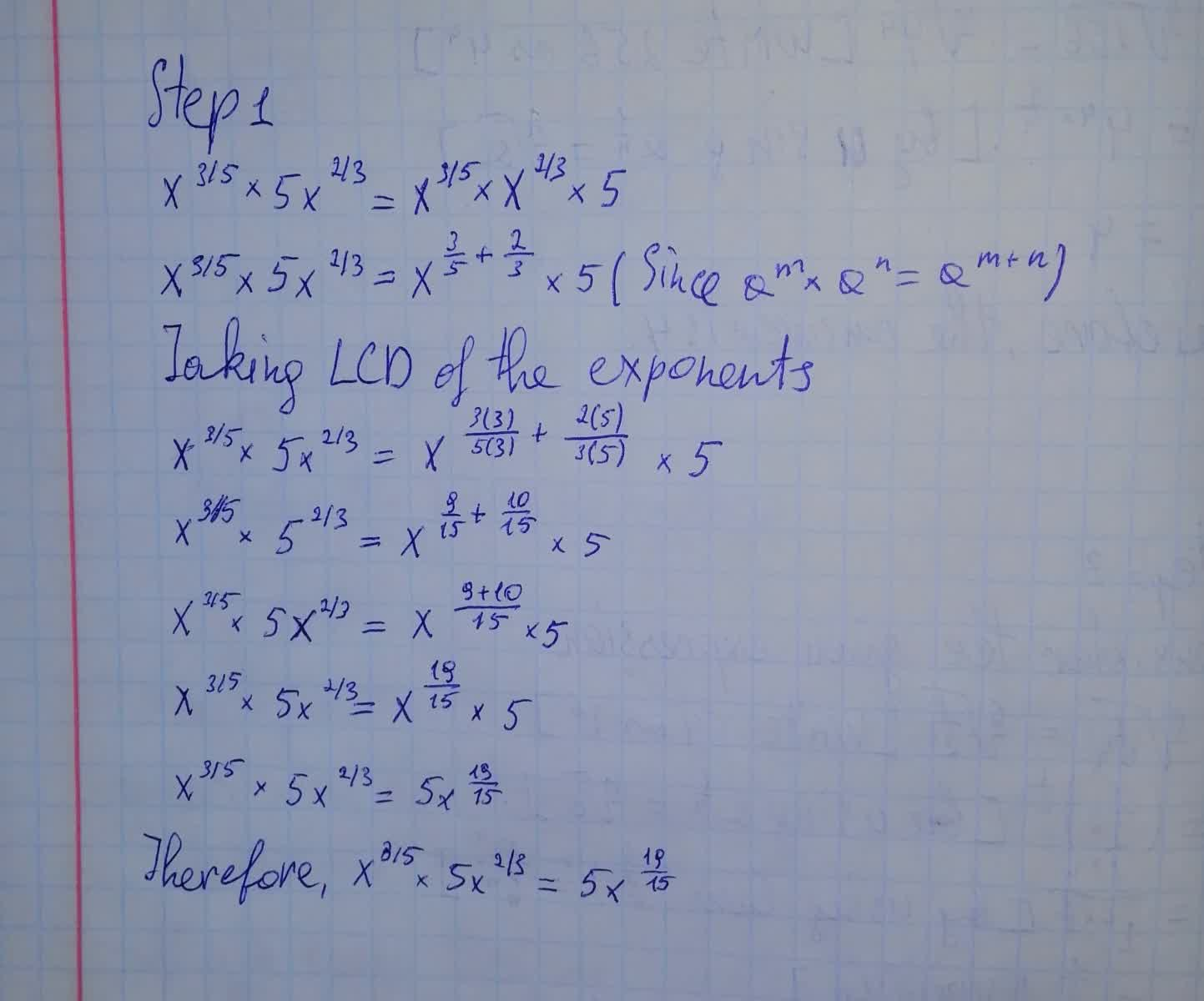# To simplify the given expression.Phoebe 2021-08-15 Answered
To simplify the given expression.You can still ask an expert for help

• Questions are typically answered in as fast as 30 minutes

Solve your problem for the price of one coffee

• Math expert for every subject
• Pay only if we can solve itbroliY
The solution is written below###### Not exactly what you’re looking for?

Rewrite using the commutative property of multiplication.

$5{x}^{\frac{3}{5}}{x}^{\frac{2}{3}}$

Multiply ${x}^{\frac{3}{5}}$ by ${x}^{\frac{2}{3}}$ by adding the exponents.

Move ${x}^{\frac{2}{3}}$

$5\left({x}^{\frac{2}{3}}{x}^{\frac{3}{5}}\right)$

Use the power rule ${a}^{m}{a}^{n}={a}^{m+n}$ to combine exponents.

To write $\frac{2}{3}$ as a fraction with a common denominator, multiply by $\frac{5}{5}$

$5{x}^{\frac{2}{3}\ast \frac{5}{5}+\frac{3}{5}}$

To write $\frac{3}{5}$ as a fraction with a common denominator, multiply by $\frac{3}{3}$

$5{x}^{\frac{2}{3}\ast \frac{5}{5}+\frac{3}{5}\ast \frac{3}{3}}$

Write each expression with a common denominator of 15, by multiplying each by an appropriate factor of 1

$5{x}^{\frac{2\ast 5}{15}+\frac{3\ast 3}{15}}$

Combine the numerators over the common denominator.

$5{x}^{\frac{2\ast 5+3\ast 3}{15}}$

Simplify the numerator.

$5{x}^{\frac{19}{15}}$ - Answer